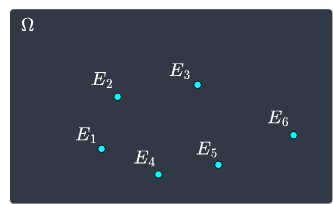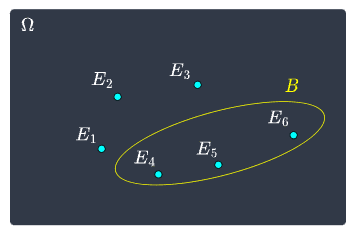search
Search
Map of Data Science
Guest 0reps
exit_to_appLog out
Map of data science
Thanks for the thanks!
close
Cancel
Post
account_circle
Profile
exit_to_app
Sign out
search
keyboard_voice
close
Searching Tips
Search for a recipe:
"Creating a table in MySQL"
Search for an API documentation: "@append"
Search for code: "!dataframe"
Apply a tag filter: "#python"
Useful Shortcuts
/ to open search panel
Esc to close search panel
to navigate between search results
d to clear all current filters
Enter to expand content previewDoc SearchCode Search BetaSORRY NOTHING FOUND!
mic
Start speaking...Voice search is only supported in Safari and Chrome.
Shrink
Navigate to
A
A
brightness_medium
share
arrow_backShareTwitterFacebook
check_circle
Mark as learned
thumb_up
2
thumb_down
0
chat_bubble_outline
0
auto_stories new
settings

# Sample space, events and axioms of probability

Probability and Statistics
chevron_right
Probability Theory
schedule Nov 1, 2022
Last updated
local_offer Probability and Statistics
Tags
map
Check out the interactive map of data science
Definition.

# Sample space and sample points

A sample space, often denoted by the letter $S$ or the symbol $\Omega$, is the set of all possible outcomes of a statistical experiment. Each outcome in a sample space is called a sample point.

Example.

## Sample space of tossing a coin

What is the sample space of tossing a coin:

• once?

• twice?

Solution. There are two outcomes when a coin is tossed: heads ($H$) or tails ($T$). Therefore, the sample space for tossing a coin once is $\Omega=\{H,T\}$. The sample space for tossing a coin twice is $\Omega=\{HH, HT, TH, HH\}$.

Example.

## Sample space of rolling a dice

What is the sample space of rolling a dice? How many sample points are there?

Solution. The sample space of rolling a dice is:

$$\Omega= \{1,2,3,4,5,6\}$$

The number of sample points is $6$, that is, there are $6$ possible outcomes in total.

Definition.

# Events, simple events and compound events

An event is a set of outcomes of an experiment. Events are always a subset of the sample space. A simple event has only one outcome, that is, it can only happen in one way. A compound event has two or more outcomes and it can be decomposed into simple events.

Example.

## Events of rolling a dice

What are some events associated with a single roll of a dice?

Solution. Here is a list of some events that may be of interest:

• event $A$ - observe an odd number, that is, $\{1,3,5\}$.

• event $B$ - observe a number greater than $3$, that is, $\{4,5,6\}$.

• event $C$ - observe a $1$, that is, $\{1\}$.

• event $D$ - observe a $6$, that is, $\{6\}$.

Here, event $A$ occurs when we observe a $1$, $3$ or $5$. Since multiple outcomes satisfy event $A$, it is considered to be a compound event. The same can be said for event $B$.

On the other hand, events $C$ and $D$ are called simple events because they cannot be decomposed, that is, there is only one way in which these events happen. We sometimes denote simple events using the letter $E$ - for instance:

• simple event $E_1$ - observe a $1$.

• simple event $E_2$ - observe a $2$.

• and so on.

Notice that any event, whether it be compound or simple, is a subset of the sample space:

$$\Omega=\{1,2,3,4,5,6\}$$

Just like for sets, we can visualize events using a Venn diagram:Recall that the compound event $B$ occurs when we observe a number greater than $3$, that is:

$$B=\{E_4,E_5,E_6\} \;\;\;\;\;\;\text{or}\;\;\;\;\;\; B=E_4\cup{E_5}\cup{E_6}$$

We can visualize event $B$ with a Venn diagram:Example.

## Tossing a coin

Suppose we toss a coin three times. What are some examples of events, simple events and compound events?

Solution. Some examples of events we might be interested in are:

• event $A$ - getting all heads.

• event $B$ - getting exactly one tails.

• event $C$ - getting more than one tails.

• event $D$ - getting at least two heads.

Note the following:

• event $A$ is a simple event because there is only one way in which this event occurs $(\mathrm{HHH})$.

• event $B$ is a compound event because this outcome can occur in multiple ways $\mathrm{TTH}$, $\mathrm{HTH}$ or $\mathrm{HHT}$.

• events $C$ and $D$ are also compound events. For instance, event $C$ occurs when we either get two or three tails.

Definition.

# Mutually exclusive events

If two events are mutually exclusive or disjoint, then they cannot happen simultaneously.

Example.

## Mutually exclusive events of rolling a dice

Suppose we roll a dice once. Let's define the following events:

• event $A$ - getting an even number.

• event $B$ - getting an odd number.

These events are mutually exclusive because they cannot occur at the same time.

Example.

## All simple events are mutually exclusive

Simple events are those that have only one outcome, that is, they can only happen in one way. For example, the simple events of tossing a coin are:

• event $A$ - getting a heads.

• event $B$ - getting a tails.

Since we cannot get a heads and tails at the same time, these simple events must be mutually exclusive.

Definition.

# Formal definition of probability based on axioms

Let $\Omega$ be the sample space associated with a statistical experiment. To every event $A$ of $\Omega$, we assign a number $\mathbb{P}(A)$ called the probability of $A$ such that the following three axioms hold:

Axiom 1: $\mathbb{P}(A)\ge0$.

Axiom 2: $\mathbb{P}(\Omega)=1$.

Axiom 3: If $A_1,A_2,A_3,\cdots$ are disjoint events, then:

$$\mathbb{P}(A_1\cup{A_2}\cup{A_3}\cup{\cdots}) =\mathbb{P}(A_1)+\mathbb{P}(A_2)+\mathbb{P}(A_3)+\cdots$$

Intuition. The first two axioms of probability are straight-forward. Axiom 1 states that the probability of an event cannot be negative. If $\mathbb{P}(A)=0$, then the event cannot occur.

Axiom 2 states that the probability of the sample space $\Omega$ is equal to one, that is, we must observe an outcome contained in the sample space. This should make sense because the sample space by definition must contain all possible outcomes, and so one of the outcomes in the sample space must occur.

Axiom 3 is much less intuitive. This axiom states that if events are disjoint or mutually exclusive, then the probability of their union must be equal to the sum of the probabilities of each event. For example, suppose we roll a fair dice once. The sample space is $\Omega=\{1,2,3,4,5,6\}$. Let's define events $E_1$ and $E_2$ as follows:

• event $E_1$ - observe a $1$.

• event $E_2$ - observe a $2$.

These events are mutually exclusive, that is, they cannot happen at the same time. Axiom 3 states that the probability that either events $E_1$ or $E_2$ happens is the sum of the probabilities of each event:

$$\begin{equation}\label{eq:Z16fxJ6ncu1YbNls3cu} \mathbb{P}(E_1\cup{E_2})= \mathbb{P}(E_1)+\mathbb{P}(E_2) \end{equation}$$

Let's understand what this intuitively means. For a fair dice, events $E_1$ and $E_2$ each occur with a probability of $1/6$. The probability that either $E_1$ or $E_2$ occurs should be the sum of their probabilities:

$$\mathbb{P}(E_1\cup{E_2})= \frac{1}{6}+\frac{1}{6}=\frac{1}{3}$$

What we have done here is applied the third axiom of probability! Similarly, we can use the third axiom for calculating the probability of rolling an odd number:

$$\mathbb{P}(E_1\cup{E_3}\cup{E_5})= \frac{1}{6}+\frac{1}{6}+\frac{1}{6}=\frac{1}{2}$$

In this way, all three axioms of probability make a lot of sense!

Definition.

# Independent events

Two events are said to be independent if the occurrence (or non-occurrence) of one event does not affect the probability that the other event will occur.

Example.

## Coins toss

Suppose we toss a coin twice and we define the following events:

• event $A$ - obtaining a heads for the first toss.

• event $B$ - obtaining a heads for the second toss.

Because the outcome of the first coin toss does not affect the outcome of the next coin toss, these events are independent.